### Composing formulas

You can create a formula or edit an existing one using Quattro Pro’s Formula Composer.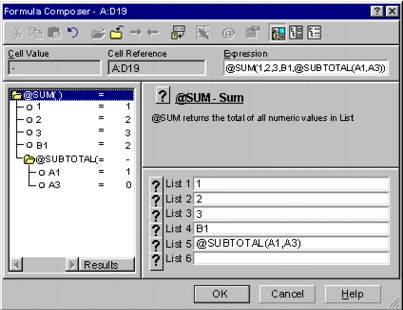This is the formula composer.

Depending on the view you choose, you can see up to three panes that provide information about the formula you are creating:

 • Outline pane — lets you examine the structure of a formula, edit parts of the formula, and trace cell references and cell names. The outline pane shows the hierarchy of the formula you have created. You can expand or collapse parts of the formula to focus on part of the formula. The outline can consist of several levels, with each level containing a different expression.
 • Spreadsheet function description pane — provides a brief description of the spreadsheet function you have selected in the outline pane. When you type a spreadsheet function (for example, @SUM) in the Expression edit field, the spreadsheet function description pane appears to the right of the dialog box.
 • Argument pane — provides entry fields for the spreadsheet function arguments. Parentheses around an argument indicate an optional argument. When you type a value for an optional argument, you must also enter values for all preceding optional arguments. Clicking to the left of an argument gives you a short description of each spreadsheet function argument.

For a list of keyboard shortcuts available in the Formula Composer dialog, see “Formula Composer keys.”

#### To create a formula in the Formula Composer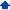1 Select a blank cell.
 2 Click the Formula Composer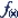button.
 3 Enable one of the following options:
 • Standard view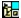— displays all three panes (spreadsheet function description, outline, and argument)
 • Argument view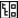— displays the outline and argument panes
 • Outline view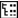— displays only the outline pane
 4 Type a formula in the Expression box.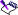• If an formula is incomplete or syntactically incorrect (for example, a spreadsheet function may be missing an argument), a red question mark icon in the outline alerts you to the error.
 • Expressions that cannot be expanded, such as values, display a small yellow circle to their left.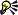• You can expand and collapse selected parts of the formula outline by clicking Expand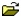and Collapse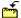.

#### To edit a formula in the Formula Composer1 Select a cell containing a formula.
 2 Click the Formula Composerbutton.
 3 Select the first expression in the outline on the left.
 4 In the Expression box, make changes to the formula.• To edit only part of a formula, select another, lower expression in the outline.